Friday , August 19 2022# NCERT 5th Class (CBSE) Mathematics: Fractions

## USING A NUMBER LINE

You can use a number line to show division of a whole number by a fraction.

(a) 5÷2/3 = ?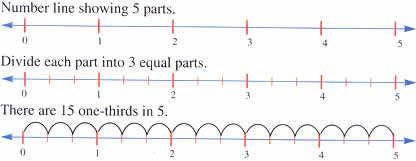5÷1/3 = 15

(b) 2÷2/5 = ?

Number line showing 5 equal parts for each number.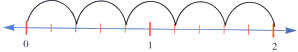There are 5 two-fifths in 2.

2÷2/5 = 5

### Dividing with Mixed Numbers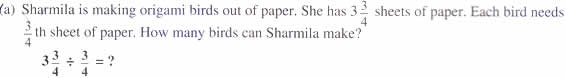####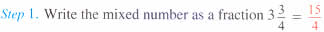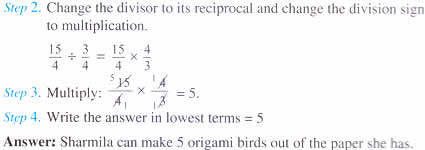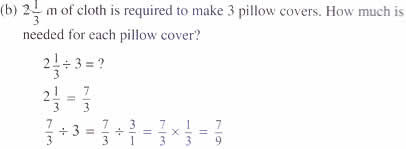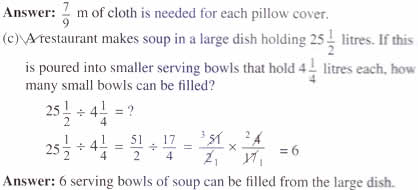1.